# Trigonometric Identities: Definition & Uses

Lesson Transcript
Instructor: Yuanxin (Amy) Yang Alcocer

Amy has a master's degree in secondary education and has been teaching math for over 9 years. Amy has worked with students at all levels from those with special needs to those that are gifted.

Trigonometric identities are equations that are always true for trigonometric functions. Learn about the definition and kinds of trigonometric identities and explore the uses of trigonometric identities through examples. Updated: 10/20/2021

## Trigonometric Identities

This video lesson is about trigonometric identities. These are the true statements about trigonometric functions. You can think of these as definitions, if you will. They explain trig functions by using simpler trig terms.

Just like there are many definitions in the English language, there are many identities in the trig world. A simple math identity is 4 = 3 + 1. In trigonometry, a simple identity can be tangent = sine/cosine. Notice that both statements are true. Both have also been written in simpler math terms.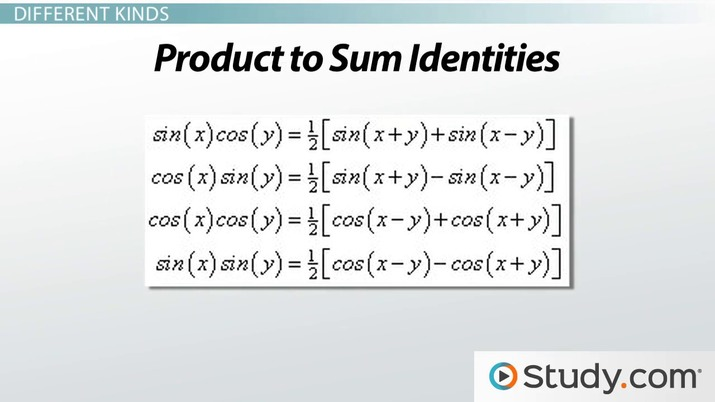An error occurred trying to load this video.

Try refreshing the page, or contact customer support.

Coming up next: Alternate Forms of Trigonometric Identities

### You're on a roll. Keep up the good work!

Replay
Your next lesson will play in 10 seconds
• 0:03 Trigonometric identities
• 0:35 Different Kinds
• 3:15 Example
• 3:48 Lesson Summary
Save Save

Want to watch this again later?

Timeline
Autoplay
Autoplay
Speed Speed

## Different Kinds

Do you remember how I said that there are all kinds of trigonometric identities, just like there are all kinds of definitions? Well, just like we can group our English words into categories, such as nouns, verbs, and adverbs, we can cluster our trigonometric identities into groups.

And just like some words in the English language are more popular, some of our trigonometric identities are more commonly used. The ones that are the most common have already been categorized into seven well-known groups. Do you want to see what they are? I'll show you along with the identities themselves:

First we have the basic identities. These are your basic definitions of your six trig functions: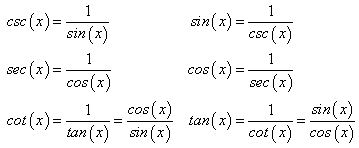The second group is called the Pythagorean identities. These are called Pythagorean because they make use of the Pythagorean theorem, which says that for a right triangle a^2 + b^2 = c^2, where c is the hypotenuse and a and b are the legs: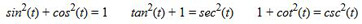The next group is called the angle sum and difference identities. These tell you how you can break down a trig function that involves the addition or subtraction of two angles: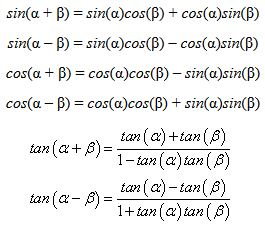The fourth group is called the double-angle identities. These tell you how you can simplify a trig function where the angle is doubled: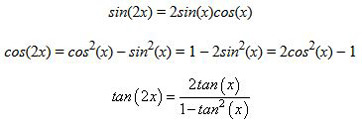The fifth group is called the half-angle identities. Similar to the double-angle identities, these tell you how you can simplify a trig function where your angle has been halved: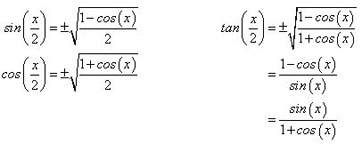For the sixth group, we have what is called the sum to product identities. These identities show you how to convert the sum or difference of two trig functions into the product of two trig functions: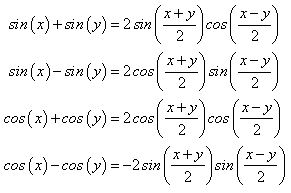The seventh and last group is called the product to sum identities. Similar to the previous group, these show how you can go from the product of two trig functions to the sum or difference of two trig functions: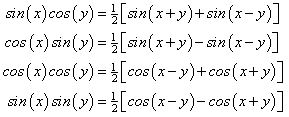What you have seen so far is just a small sampling of all the trig identities out there. But, if you can memorize these, then you are well on your way to becoming a trig expert because these are the most commonly used identities in trigonometry.

To unlock this lesson you must be a Study.com Member.

### Register to view this lesson

Are you a student or a teacher?